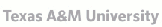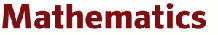## Texas Algebraic Geometry Symposium

Texas A&M University 30 September – 2 October 2022.

### AbstractsTEXAS A&M UNIVERSITY College of Arts & Sciences
 Jack Jeffries Are determinantal rings direct summands of polynomial rings? Over any infinite field, the coordinate rings of determinantal varieties are known to be fixed subrings of the action of the general linear group on a polynomial ring. This, plus the fact that the general linear group is linearly reductive in characteristic zero, explains many of their good algebraic and geometric properties in this case. In positive characteristic, these determinantal rings have many of the same good properties even though the general linear group is no longer linearly reductive. In this talk we investigate if these determinantal rings continue to be direct summands of polynomial rings in characteristic p>0. We will also encounter some interesting varieties related to linear algebra along the way. Brandon Levin Moduli of Galois representations and Serre's modularity conjecture Serre's modularity conjecture (1973) was proven by Khare-Wintenberger building on work of Kisin in the mid-2000s. The methods and problems surrounding the conjecture have played an important role in the field of modularity lifting and the p-adic Langlands program. One such development is the recent construction of moduli stacks of p-adic Galois representation by Emerton–Gee. I will explain the connection between Emerton--Gee's space and Serre's conjecture in the simplest case of two-dimensional representation of the Galois group of Qp. I will then go on to describe how this geometry helps us understand higher dimensional generalizations of Serre's conjecture based on joint work with Daniel Le, Bao V. Le Hung, and Stefano Morra. Aida Maraj Colored Gaussian Graphical Models with Toric Vanishing Ideals? A Colored Gaussian graphical model is a collection of multivariate Gaussian distributions in which a colored graph encodes conditional independence relations among the random variables. Its set of concentration matrices is a linear space of symmetric matrices intersected with the cone of positive definite matrices. Its inverse space, the space of covariance matrices, on the contrary, most often is not a friendly variety. We are interested in the equations that vanish on the covariance matrices of these models. The main result of the talk is that colored Gaussian graphical models with block graph structure that satisfy certain permutation symmetries (RCOP) are toric in the space of covariance matrices. We provide explicit Markov bases/generating sets for the vanishing ideal of this variety which can be read from paths in the graph. The talk is based on the preprint arXiv:2111.14817. Patricia Klein Alternating sign matrix varieties Matrix Schubert varieties, introduced by Fulton in the 1990s, are affine varieties that ``live above'' Schubert varieties in the complete flag variety. They have many desirable algebro-geometric properties, such as irreducibility, Cohen–Macaulayness, and easily-computed dimension. They also enjoy a close connection with the symmetric groups. Alternating sign matrix (ASM) varieties, introduced by Weigandt just several years ago, are generalizations of matrix Schubert varieties in two senses: (1) ASM varieties are unions of matrix Schubert varieties and (2) the defining equations of ASM varieties are determined by ASMs, which are generalizations of permutation matrices. ASMs have been important objects of study in enumerative combinatorics since at least the 1980s and appear in statistical mechanics as the 6-vertex lattice model. Although ASMs have a robust combinatorial underpinning and although their irreducible components are matrix Schubert varieties, they are nevertheless much more difficult to get a handle on than matrix Schubert varieties themselves. In this talk, we will define ASMs, compare and contrast with matrix Schubert varieties, and state some open problems. Evangelia Gazaki Local-to-global principles for zero-cycles Let X be a smooth projective variety over the rational numbers. The set X(Q) of all rational points of X embeds to the set of all real points, as well as to the set of all p-adic points, for every prime number p. The classical local-to-global principles for X refer to the diagonal embedding of X(Q) to the direct product of the above sets. We say that X satisfies Weak Approximation, if the image of this embedding is dense. When this is not the case, one looks for possible obstructions. A classical obstruction is given by the Brauer group of X. This obstruction is expected to explain all phenomena for certain classes of varieties, but it is generally not the only one. In this talk we will discuss analogs of these questions for the Chow group CH0(X) of zero-cycles on X. In this case, there is a very open conjecture due to Colliot-Thelene and Sansuc and separately due to Kato and Saito that the obstruction induced by the Brauer group is the only one. We will give evidence for this conjecture for a product of elliptic curves. This is based on joint work with T. Hiranouchi, and a preprint with an Appendix by A. Koutsianas. Patricio Gallardo /Moduli of points in the plane, wall-crossings, and non-reduced schemes A central insight of algebraic geometry is that a moduli space has many geometrically meaningful compactifications. Moreover, much of their birational geometry can be understood via the degenerations these compactifications are parametrizing. Describing such interplay is one of the leading questions in moduli theory nowadays. Within this context, we describe joint work with Benjamin Schmidt on the moduli of unlabelled points in the plane. Our spaces are obtained via GIT quotients of the Hilbert scheme and its birational models constructed with Bridgeland stability. In particular, we describe the role of non-reduced schemes on the induced wall and chamber decomposition within the movable cone of the Hilbert scheme. Bernd Siebert Toward the logarithmic Hilbert scheme Logarithmic geometry provides tools to work relative a normal crossings divisor, including normal crossings degenerations. I will report on work in progress with Mattia Talpo and Richard Thomas to define a natural logarithmic analogue of the ordinary Hilbert scheme. Immediate applications include induced good degenerations of Hilbert schemes of points. Our point of view also suggests a definition of tropical Hilbert schemes. Jesse Kass Counting curves arithmetically A basic subject of research in algebraic geometry is counting curves in special position: how many lines on a plane cubic surface (27), how many rational curves passing through 11 general points (620), etc. The focus has been on counting curves over the complex numbers, or more generally an algebraically closed field. Generalizing work on counting curves over the real numbers, my talk will discuss how to prove results over fields that are more arithmetically interesting, like a finite field or the p-adic numbers. All orignal results are joint with Marc Levine, Jake Solomon, and Kirsten Wickelgren.